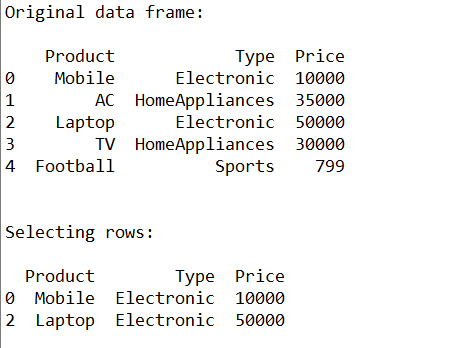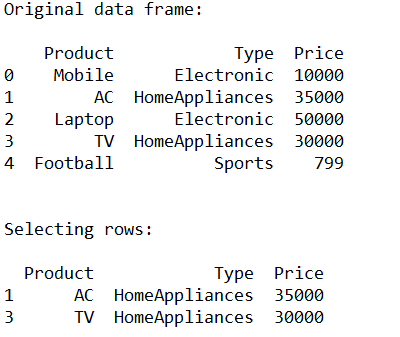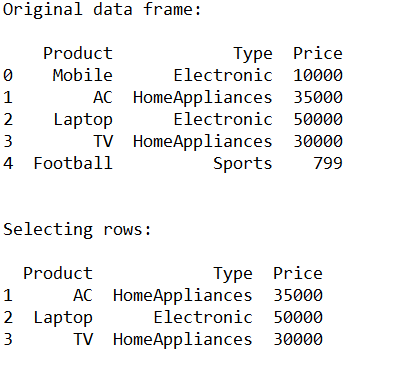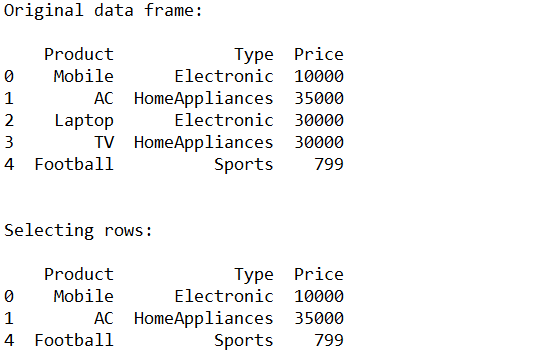# How to Select Rows from Pandas DataFrame?

• Last Updated : 10 Jul, 2020

`pandas.DataFrame.loc` is a function used to select rows from Pandas DataFrame based on the condition provided. In this article, let’s learn to select the rows from Pandas DataFrame based on some conditions.

Syntax: df.loc[df[‘cname’] ‘condition’]

Attention geek! Strengthen your foundations with the Python Programming Foundation Course and learn the basics.

To begin with, your interview preparations Enhance your Data Structures concepts with the Python DS Course. And to begin with your Machine Learning Journey, join the Machine Learning - Basic Level Course

Parameters:
df: represents data frame
cname: represents column name
condition: represents condition on which rows has to be selected

Example 1:

 `# Importing pandas as pd``from` `pandas ``import` `DataFrame`` ` `# Creating a data frame``cart ``=` `{``'Product'``: [``'Mobile'``, ``'AC'``, ``'Laptop'``, ``'TV'``, ``'Football'``],``        ``'Type'``: [``'Electronic'``, ``'HomeAppliances'``, ``'Electronic'``, ``                 ``'HomeAppliances'``, ``'Sports'``],``        ``'Price'``: [``10000``, ``35000``, ``50000``, ``30000``, ``799``]``       ``}`` ` `df ``=` `DataFrame(cart, columns ``=` `[``'Product'``, ``'Type'``, ``'Price'``])`` ` `# Print original data frame``print``(``"Original data frame:\n"``)``print``(df)`` ` `# Selecting the product of Electronic Type``select_prod ``=` `df.loc[df[``'Type'``] ``=``=` `'Electronic'``]`` ` `print``(``"\n"``)`` ` `# Print selected rows based on the condition``print``(``"Selecting rows:\n"``)``print` `(select_prod)`

Output:Example 2:

 `# Importing pandas as pd``from` `pandas ``import` `DataFrame`` ` `# Creating a data frame``cart ``=` `{``'Product'``: [``'Mobile'``, ``'AC'``, ``'Laptop'``, ``'TV'``, ``'Football'``],``        ``'Type'``: [``'Electronic'``, ``'HomeAppliances'``, ``'Electronic'``,``                 ``'HomeAppliances'``, ``'Sports'``],``        ``'Price'``: [``10000``, ``35000``, ``50000``, ``30000``, ``799``]``       ``}`` ` `df ``=` `DataFrame(cart, columns ``=` `[``'Product'``, ``'Type'``, ``'Price'``])`` ` `# Print original data frame``print``(``"Original data frame:\n"``)``print``(df)`` ` `# Selecting the product of HomeAppliances Type``select_prod ``=` `df.loc[df[``'Type'``] ``=``=` `'HomeAppliances'``]`` ` `print``(``"\n"``)`` ` `# Print selected rows based on the condition``print``(``"Selecting rows:\n"``)``print` `(select_prod)`

Output:Example 3:

 `# Importing pandas as pd``from` `pandas ``import` `DataFrame`` ` `# Creating a data frame``cart ``=` `{``'Product'``: [``'Mobile'``, ``'AC'``, ``'Laptop'``, ``'TV'``, ``'Football'``],``        ``'Type'``: [``'Electronic'``, ``'HomeAppliances'``, ``'Electronic'``,``                 ``'HomeAppliances'``, ``'Sports'``],``        ``'Price'``: [``10000``, ``35000``, ``50000``, ``30000``, ``799``]``       ``}`` ` `df ``=` `DataFrame(cart, columns ``=` `[``'Product'``, ``'Type'``, ``'Price'``])`` ` `# Print original data frame``print``(``"Original data frame:\n"``)``print``(df)`` ` `# Selecting the product of Price greater ``# than or equal to 25000``select_prod ``=` `df.loc[df[``'Price'``] >``=` `25000``]`` ` `print``(``"\n"``)`` ` `# Print selected rows based on the condition``print``(``"Selecting rows:\n"``)``print` `(select_prod)`

Output:Example 4:

 `# Importing pandas as pd``from` `pandas ``import` `DataFrame`` ` `# Creating a data frame``cart ``=` `{``'Product'``: [``'Mobile'``, ``'AC'``, ``'Laptop'``, ``'TV'``, ``'Football'``],``        ``'Type'``: [``'Electronic'``, ``'HomeAppliances'``, ``'Electronic'``,``                 ``'HomeAppliances'``, ``'Sports'``],``        ``'Price'``: [``10000``, ``35000``, ``30000``, ``30000``, ``799``]``       ``}`` ` `df ``=` `DataFrame(cart, columns ``=` `[``'Product'``, ``'Type'``, ``'Price'``])`` ` `# Print original data frame``print``(``"Original data frame:\n"``)``print``(df)`` ` `# Selecting the product of Price not ``# equal to 30000``select_prod ``=` `df.loc[df[``'Price'``] !``=` `30000``]`` ` `print``(``"\n"``)`` ` `# Print selected rows based on the condition``print``(``"Selecting rows:\n"``)``print` `(select_prod)`

Output:My Personal Notes arrow_drop_up